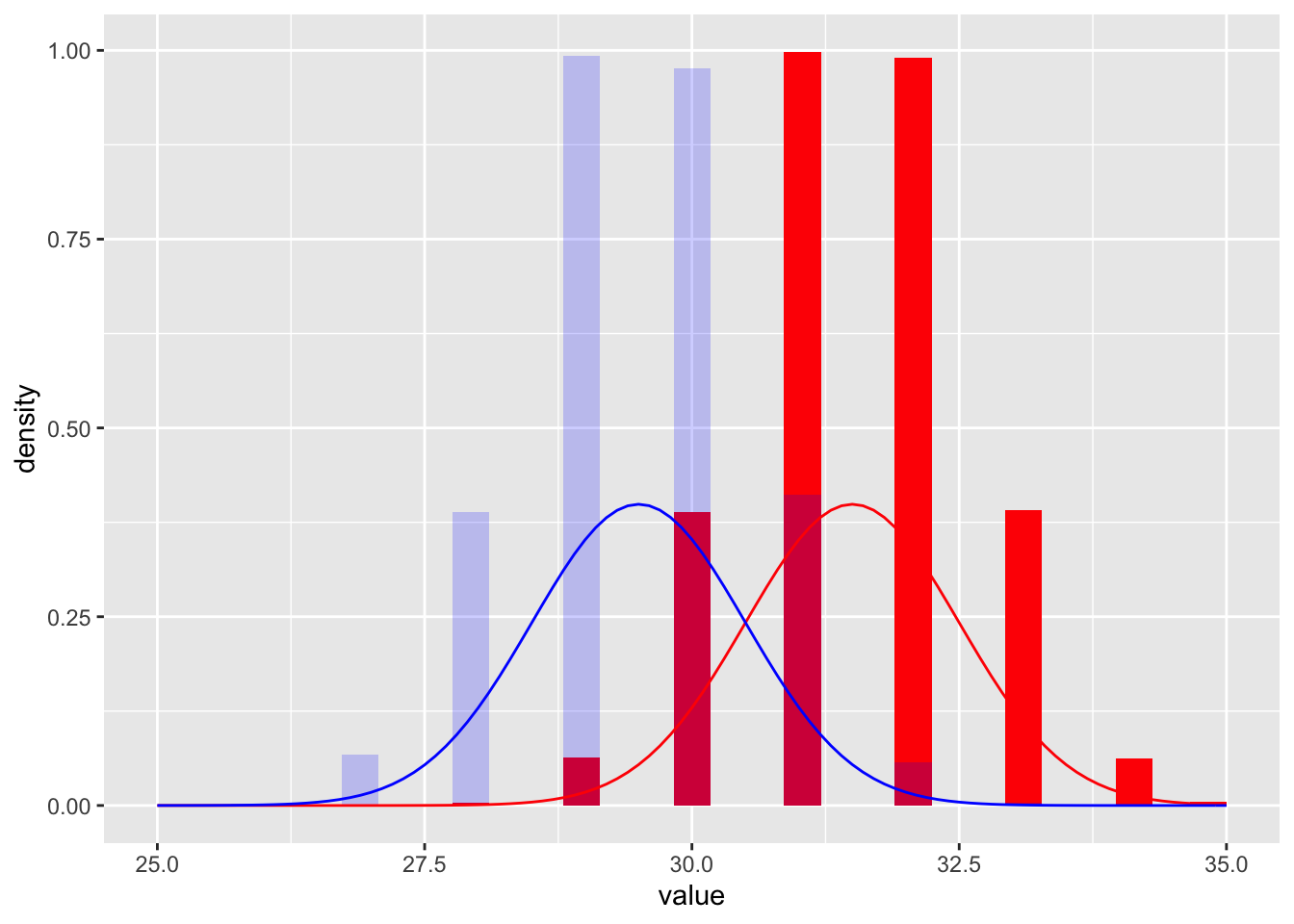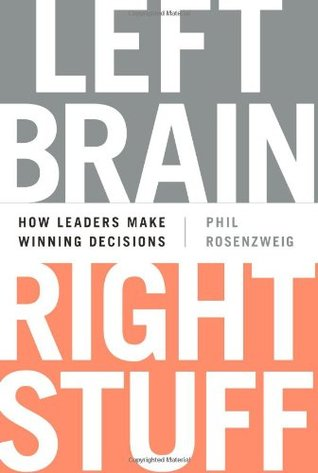# Richard Sprague

My personal website

# Monte Carlo and Team Performance in R

### 2018-04-15

Monte Carlo simulations are a simple, though computationally-expensive, way to calculate probabilities when the underlying parameters are too complicated to represent in a simple equation. Here’s a simple example.

Imagine two teams, A and B, competing against each other in a competition to see who can score the most number of points. Team A is slightly better, usually getting about 32 points on average, whereas Team B usually gets about 30. Assuming that the good days and bad days for each team follow a normal distribution, what percentage of the time can we expect the stronger team (A) to beat the weaker (B)?

Here are 10,000 simulations for each team.

``````plays <- 10000

a <- as.integer(rnorm(plays,32))
b <- as.integer(rnorm(plays,30))

games <- data.frame(trial=1:plays,a,b)

games.df <- games %>% gather(trial,"value")
ggplot(data=games.df,aes(x=value)) +
geom_histogram(data=dplyr::filter(games.df,trial=="a"), fill="red", aes(y=..density.., fill = ..count..)) +
geom_histogram(data=dplyr::filter(games.df,trial=="b"), fill="blue", alpha=0.2, aes(y=..density.., fill = ..count..)) +
stat_function(fun = dnorm, n = 101, color="red", args = list(mean = mean(dplyr::filter(games.df,trial=="a") %>% select(value) %>% unlist()), sd = 1)) +
stat_function(fun = dnorm, n = 101, color="blue", args = list(mean = mean(dplyr::filter(games.df,trial=="b") %>% select(value) %>% unlist()), sd = 1)) ``````
``````## `stat_bin()` using `bins = 30`. Pick better value with `binwidth`.
## `stat_bin()` using `bins = 30`. Pick better value with `binwidth`.``````But if the teams are playing each other, only one can win. Obviously, Team A, being slightly better, will usually win, but not always.

Here is the percentage of games Team A will win:

``knitr::kable(data.frame(A=sum(games\$a>games\$b)*100 / plays, B=sum(games\$b>games\$a)*100 / plays , Tie=sum(games\$b==games\$a)*100/plays), digits = 2, caption = "Percentage of each outcome")``
Table 1: Percentage of each outcome
A B Tie
85.42 4.27 10.31

What’s interesting is that, although the two teams are fairly closely matched – A is only slightly better than B – in the long run the advantage is overwhelmingly in A’s favor. The lesson is if you can improve yourself even a tiny bit over what you previously thought possible, you will greatly increase your winning.

Questions about R: I wrote this as a quick-and-dirty example of solving a problem like this in R, but obviously the chart is slightly wrong. I’d like the distribution function line and the histograms to match, but I can’t quite figure out how to do that. Any hints?

Note: this example was inspired by Philip Rosenzweig’s excellent book Left Brain, Right Stuff